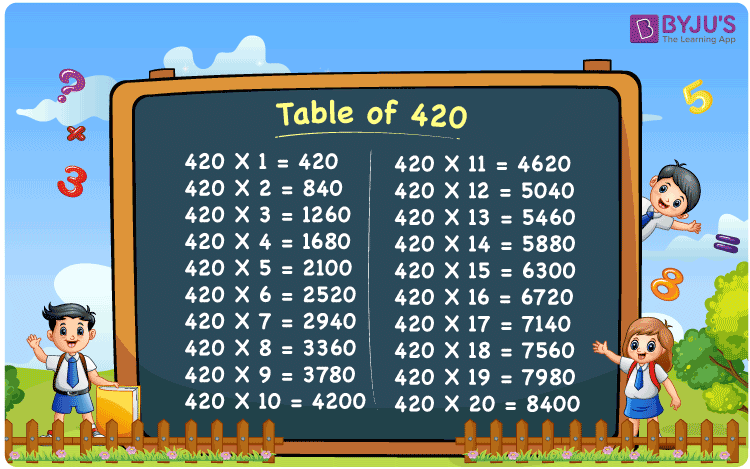Checkout JEE MAINS 2022 Question Paper Analysis : Checkout JEE MAINS 2022 Question Paper Analysis :

# Table of 420

The table of 420 is a multiplication table made up of 420 and natural numbers multiplied together. It is important to learn the 420 times table because it speeds up the completion of mathematical assignments. On this page, you will find a 420 times chart and a tabular form of the 420 multiplication table. The PDF for the table of 420 can be downloaded by clicking the link below.

## Table of 420 Chart## What is the 420 Times Table?

The below table depicts the repeated addition and multiplication table of 420.

 420×1 = 420 420 420×2 = 840 420 + 420 = 840 420×3 = 1260 420 + 420 + 420 = 1260 420×4 = 1680 420 + 420 + 420 + 420 = 1680 420×5 = 2100 420 + 420 + 420 + 420 + 420 = 2100 420×6 = 2520 420 + 420 + 420 + 420 + 420 + 420 = 2520 420×7 = 2940 420 + 420 + 420 + 420 + 420 + 420 + 420 = 2940 420×8 = 3360 420 + 420 + 420 + 420 + 420 + 420 + 420 + 420 = 3360 420×9 = 3780 420 + 420 + 420 + 420 + 420 + 420 + 420 + 420 + 420 = 3780 420×10 = 4200 420 + 420 + 420 + 420 + 420 + 420 + 420 + 420 + 420 + 420 = 4200

## Multiplication Table of 420

The table of 420 in the tabular form is given below.

 420 × 1 = 420 420 × 2 = 840 420 × 3 = 1260 420 × 4 = 1680 420 × 5 = 2100 420 × 6 = 2520 420 × 7 = 2940 420 × 8 = 3360 420 × 9 = 3780 420 × 10 = 4200 420 × 11 = 4620 420 × 12 = 5040 420 × 13 = 5460 420 × 14 = 5880 420 × 15 = 6300 420 × 16 = 6720 420 × 17 = 7140 420 × 18 = 7560 420 × 19 = 7980 420 × 20 = 8400

## Solved Example on the Table of 420

Example:

Find the missing value: 420 × ____ = 6300.

Solution:

Let the missing value be “y”.

I.e., 420y = 6300

y = 6300/420

y = 15

Hence, the missing value is 15.

I.e., 420 × 15 = 6300.

## Frequently Asked Questions on the Table of 420

### What is the table of 420?

The table of 420 is the multiplication table which is obtained from the product of 420 and natural numbers.

### Write the 420 times table.

420×1 = 420, 420×2 = 840, 420×3 = 1260, 420×4 = 1680, 420×5 = 2100, 420×6 = 2520, 420×7 = 2940, 420×8 = 3360, 420×9 = 3780, 420×10 = 4200.

### What is 420 times 10?

420 times 10 is 4200. I.e., 420 × 10 = 4200.